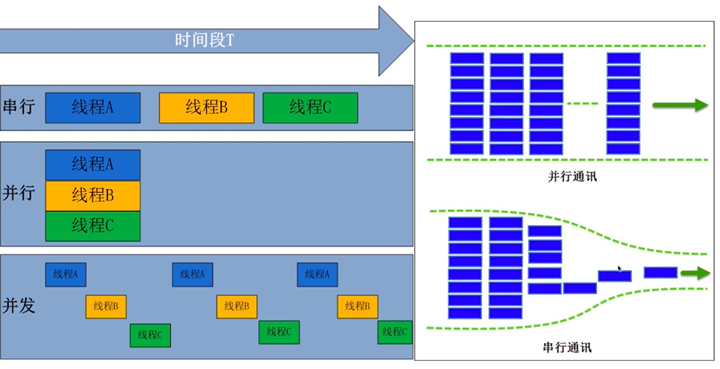# Python迭代器与生成器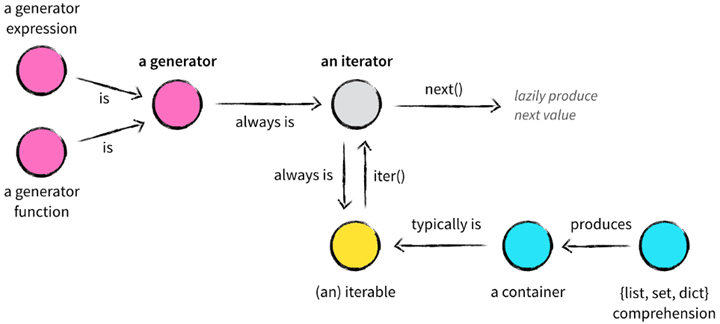## 容器（container）

• list, deque, ….
• set, frozensets, ….
• dict, defaultdict, OrderedDict, Counter, ….
• tuple, namedtuple, …
• str

## 可迭代对象(iterable)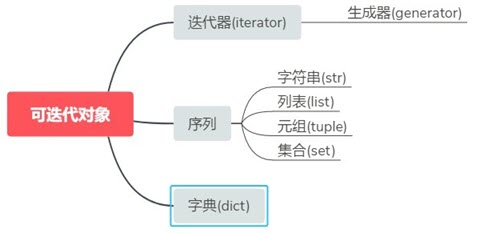from collections.abc import Iterable

def iterable_test_1(obj):
return isinstance(obj, Iterable)

def iterable_test_2(obj):
try:
iter(obj)
return True
except TypeError:
return False

def iterable_test_3(obj):
try:
for i in obj:
pass
return True
except TypeError:
return False

if __name__ == "__main__":
print(iterable_test_1("Hello World!"))
print(iterable_test_2("Hello World!"))
print(iterable_test_3("Hello World!"))
print(iterable_test_1(123))
print(iterable_test_2(123))
print(iterable_test_3(123))


## 迭代器(iterator)

• __iter__：返回迭代器本身self。
• __next__：返回迭代器下一个可用的元素，当最后没有元素时抛出StopIteration异常。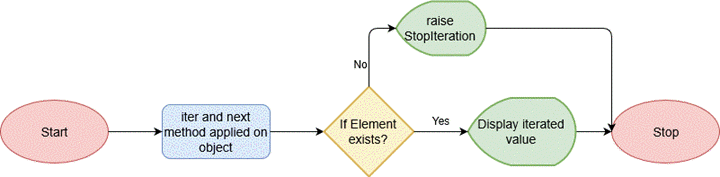• 迭代器一定是可迭代对象，因为实现了__iter__方法。
• 迭代器的__iter__方法返回的是自身，并不产生新的迭代器对象，只能遍历一次。若想再次迭代需要重建迭代器。
• 迭代器是惰性计算，只有在调用时才返回值，没有调用的时候就等待下一次调用。这样就节省了大量内存空间。

• 提供一种通用的且不依赖于索引的迭代取值方式
• 同一时刻在内存中只存在一个值，更节省内存

• 取值不如按照索引的方式灵活，（不能取指定的某一个值，而且只能往后取）
• 无法预测迭代器的长度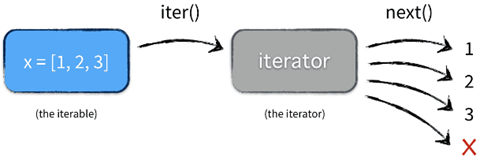# 创建一个迭代器类
class Fib:
def __init__(self):
self.prev = 0
self.curr = 1

def __iter__(self):
return self

def __next__(self):
value = self.curr
self.curr += self.prev
self.prev = value
if value > 500:
raise StopIteration
return value
print(Fib())

# 根据可迭代对象创建一个迭代器
lst = [1, 2, 3]
res = iter(lst)
print(res)

# 判断可迭代对象是否为迭代器
from collections.abc import Iterator
print(isinstance(lst, Iterator))
print(isinstance(res, Iterator))


## 生成器(generator)

• 生成器函数(generator function)
• 生成器表达式(generator expression)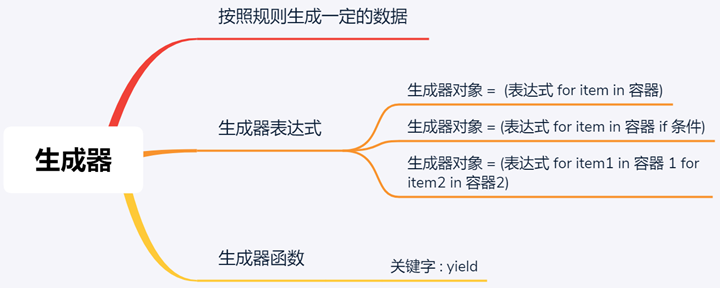### 生成器函数(generator function)

def fibonacci(n):
a, b, counter = 1, 1, 0
while counter < n:
yield a
a, b = b, a + b
counter += 1

f = fibonacci(10)
print(next(f))
print(next(f))
print('----')
for item in f:
print(item)


### 生成器表达式(generator expression)

nums_squared_lc = [num**2 for num in range(5)]
nums_squared_gc = (num**2 for num in range(5))

if __name__ == "__main__":
print(nums_squared_lc)
print(nums_squared_gc)
# 输出
# [0, 1, 4, 9, 16]
# <generator object <genexpr> at 0x0000022D4FD5EDC8>


### 生成器使用示例

csv_gen = csv_reader("some_csv.txt")
row_count = 0

for row in csv_gen:
row_count += 1

print(f"Row count is {row_count}")


def csv_reader(file_name):
file = open(file_name)
return result


Traceback (most recent call last):
File "ex1_naive.py", line 22, in <module>
main()
File "ex1_naive.py", line 13, in main
File "ex1_naive.py", line 6, in csv_reader
MemoryError


def csv_reader(file_name):
for row in open(file_name, "r"):
yield row


csv_gen = (row for row in open(file_name))

a = range(5)
print(list(a))


def infinite_sequence():
num = 0
while True:
yield num
num += 1

if __name__ == "__main__":
for i in infinite_sequence():
print(i, end=" ")


gen = infinite_sequence()
print(next(gen))


def infinite_sequence():
num = 0
while True:
yield num
num += 1

def is_palindrome(num):
# Skip single-digit inputs
if num // 10 == 0:
return False
temp = num
reversed_num = 0

while temp != 0:
reversed_num = (reversed_num * 10) + (temp % 10)
temp = temp // 10

if num == reversed_num:
return num
else:
return False

if __name__ == "__main__":
for i in infinite_sequence():
pal = is_palindrome(i)
if pal:
print(i)


### 生成器性能

nums_squared_lc = [num**2 for num in range(10000)]
nums_squared_gc = (num**2 for num in range(10000))

if __name__ == "__main__":
import sys
print(sys.getsizeof(nums_squared_lc))
print(sys.getsizeof(nums_squared_gc))
# 输出
# 87624
# 120


import cProfile
print(cProfile.run('sum([i * 2 for i in range(10000)])'))
print(cProfile.run('sum((i * 2 for i in range(10000)))'))
# 输出
#          5 function calls in 0.001 seconds
#
#    Ordered by: standard name
#
#    ncalls  tottime  percall  cumtime  percall filename:lineno(function)
#         1    0.001    0.001    0.001    0.001 <string>:1(<listcomp>)
#         1    0.000    0.000    0.001    0.001 <string>:1(<module>)
#         1    0.000    0.000    0.001    0.001 {built-in method builtins.exec}
#         1    0.000    0.000    0.000    0.000 {built-in method builtins.sum}
#         1    0.000    0.000    0.000    0.000 {method 'disable' of '_lsprof.Profiler' objects}
#
#
# None
#          10005 function calls in 0.002 seconds
#
#    Ordered by: standard name
#
#    ncalls  tottime  percall  cumtime  percall filename:lineno(function)
#     10001    0.001    0.000    0.001    0.000 <string>:1(<genexpr>)
#         1    0.000    0.000    0.002    0.002 <string>:1(<module>)
#         1    0.000    0.000    0.002    0.002 {built-in method builtins.exec}
#         1    0.001    0.001    0.002    0.002 {built-in method builtins.sum}
#         1    0.000    0.000    0.000    0.000 {method 'disable' of '_lsprof.Profiler' objects}
#
#
# None


### 高级生成器方法

• .send()
• .throw()
• .close()

def infinite_palindromes():
num = 0
while True:
if is_palindrome(num):
i = (yield num)
if i is not None:
num = i
num += 1

def is_palindrome(num):
# Skip single-digit inputs
if num // 10 == 0:
return False
temp = num
reversed_num = 0

while temp != 0:
reversed_num = (reversed_num * 10) + (temp % 10)
temp = temp // 10

if num == reversed_num:
return True
else:
return False

if __name__ == "__main__":
pal_gen = infinite_palindromes()
for i in pal_gen:
digits = len(str(i))
pal_gen.send(10 ** (digits))
print(i)


• infinite_palindromes() 中i = (yield num)，检查if i is not None，如果next()在生成器对象上调用可能会发生这种情况。
• send()，将执行 10 ** digits给i，更新num

.throw()允许您使用生成器抛出异常。在下面的示例中，此代码将在抛出digits=5时抛出ValueError：

if __name__ == "__main__":
pal_gen = infinite_palindromes()
for i in pal_gen:
print(i)
digits = len(str(i))
if digits == 5:
pal_gen.throw(ValueError("We don't like large palindromes"))
pal_gen.send(10 ** (digits))
print(i)


.throw()在您可能需要捕获异常的任何领域都很有用。

if __name__ == "__main__":
pal_gen = infinite_palindromes()
for i in pal_gen:
print(i)
digits = len(str(i))
if digits == 5:
pal_gen.close()
pal_gen.send(10 ** (digits))
print(i)


.close()是它引发了StopIteration一个异常，用于表示有限迭代器的结束。

### 使用生成器创建数据管道

file_name = "techcrunch.csv"
lines = (line for line in open(file_name))
list_line = (s.rstrip().split(",") for s in lines)
cols = next(list_line)
company_dicts = (dict(zip(cols, data)) for data in list_line)
funding = (int(company_dict["raisedAmt"]) for company_dict in company_dicts if company_dict["round"] == "a")
total_series_a = sum(funding)
print(f"Total series A fundraising: \${total_series_a}")


## for循环工作机制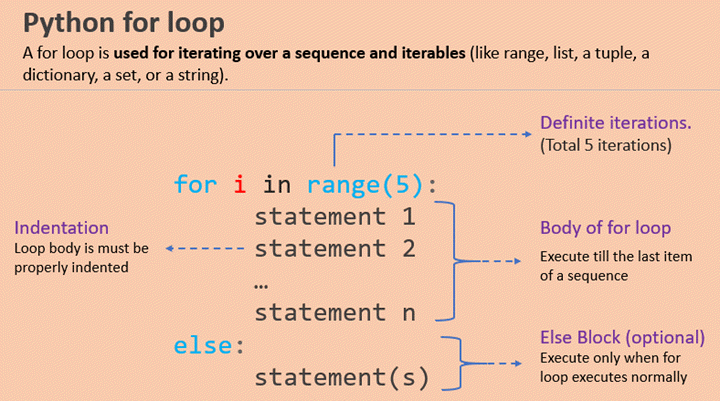• 先判断对象是否为可迭代对象(等价于判断有没有iter或getitem方法)，如果不可迭代则抛出TypeError异常。如果为可迭代对象则调用__iter__方法，返回一个迭代器。
• 不断调用迭代器的__next__方法，每次按序返回迭代器中的一个值。
• 迭代到最后没有元素时，就抛出异常 StopIteration。这个异常Python自己会处理，不会暴露给开发者。

• 调用__iter__方法，返回自身self，也就是返回迭代器。
• 不断调用迭代器的next()方法，每次按序返回迭代器中的一个值。
• 迭代到最后没有元素时，就抛出异常 StopIteration。

• 如果对象定义了__iter__，则会返回一个迭代器。
• 如果对象没有定义__iter__，但是实现了__getitem__，会改用下标迭代的方式。

## 标准库中的生成器函数

 模块 函数 说明 itertools compress(it, selector_it) 并行处理两个可迭代对象。若 selector_it 中的元素是真值，产出 it 中对应的元素 itertools dropwhile(predicate, it) 把可迭代对象 it 中的元素传给 predicate，跳过 predicate(item) 为真值的元素，在 predicate(item) 为假时停止，产出剩余（未跳过）的所有元素（不再继续检查） 内置 filter(predicate, it) 把 it 中的各个元素传给 predicate，若 predicate(item) 返回真值，产出对应元素 itertools filterfalse(predicate, it) 与 filter 函数类似，不过 predicate(item) 返回假值时产出对应元素 itertools takewhile(predicate, it) predicate(item) 返回真值时产出对应元素，然后立即停止不再继续检查 itertools islice(it, stop) 或 islice(it, start, stop, step=1) 产出 it 的切片，作用类似于 s[:stop] 或 s[start:stop:step，不过 it 可以是任何可迭代对象，且实现的是惰性操作

 模块 函数 说明 itertools accumulate(it, [func]) 产出累积的总和。若提供了 func，则把 it 中的前两个元素传给 func，再把计算结果连同下一个元素传给 func，以此类推，产出结果 内置 enumerate(it, start=0) 产出由两个元素构成的元组，结构是 (index, item)。其中 index 从 start 开始计数，item 则从 it 中获取 内置 map(func, it1, [it2, …, itN]) 把 it 中的各个元素传给 func，产出结果；若传入 N 个可迭代对象，则 func 必须能接受 N 个参数，且并行处理各个可迭代对象

 模块 函数 说明 itertools chain(it1, …, itN) 先产出 it1 中的所有元素，然后产出 it2 中的所有元素，以此类推，无缝连接 itertools chain.from_iterable(it) 产出 it 生成的各个可迭代对象中的元素，一个接一个无缝连接；it 中的元素应该为可迭代对象（即 it 是嵌套了可迭代对象的可迭代对象） itertools product(it1, …, itN, repeat=1) 计算笛卡尔积。从输入的各个可迭代对象中获取元素，合并成 N 个元素组成的元组，与嵌套的 for 循环效果一样。repeat 指明重复处理多少次输入的可迭代对象 内置 zip(it1, …, itN) 并行从输入的各个可迭代对象中获取元素，产出由 N 个元素组成的元组。只要其中任何一个可迭代对象到头了，就直接停止 itertools zip_longest(it1, …, itN, fillvalue=None) 并行从输入的各个可迭代对象中获取元素，产出由 N 个元素组成的元组，等到最长的可迭代对象到头后才停止。空缺的值用 fillvalue 填充

 模块 函数 说明 itertools groupby(it, key=None) 产出由两个元素组成的元素，形式为 (key, group)，其中 key 是分组标准，group 是生成器，用于产出分组里的元素 内置 reversed(seq) 从后向前，倒序产出 seq 中的元素；seq 必须是序列，或者实现了 __reversed__ 特殊方法的对象 itertools tee(it, n=2) 产出一个有 n 个生成器组成的元组，每个生成器都可以独立地产出输入的可迭代对象中的元素

 模块 函数 说明 内置 all(it) it 中的所有元素都为真值时返回 True，否则返回 False；all([]) 返回 True 内置 any(it) 只要 it 中有元素为真值就返回 True，否则返回 False；any([]) 返回 False 内置 max(it, [key=], [default=]) 返回 it 中值最大的元素；key 是排序函数，与 sorted 中的一样；若可迭代对象为空，返回 default 内置 min(it, [key=], [default=]) 返回 it 中值最小的元素；key 是排序函数；若可迭代对象为空，返回 default functools reduce(func, it, [initial]) 把前两个元素传给 func，然后把计算结果和第三个元素传给 func，以此类推，返回最后的结果。若提供了 initial，则将其作为第一个元素传入 内置 sum(it, start=0) it 中所有元素的总和，若提供可选的 start，会把它也加上##### 2022，还值得学习PHP吗？##### 如何理解Python装饰器